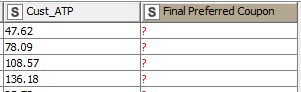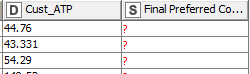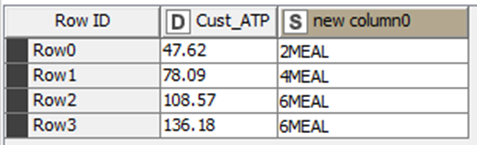# Column Expression or Rule Engine Functionality

Hi,

I have recently started using knime and i’m stuck with a problem where, i want to append a column based on a rule. However when i use column expression with script it doesn’t give the value that i’m mentioning in the formula.
So, I want to check column name “Cust_APT” which is a string and then give me the value that I define. (attaching the screenshot) which for some reason it is not working.And the script that I’m using is below -

else if ((column(“Cust_ATP”) > 95) == “TRUE”) {“6MEAL”}
else if ((column(“Cust_ATP”) >= 65) & (column(“Cust_ATP”) < 75) == “TRUE”) {“EVM”}
else if ((column(“Cust_ATP”) >= 75) & (column(“Cust_ATP”) < 95) == “TRUE”) {“4MEAL”}
else if ((column(“Cust_ATP”) >= 50) & (column(“Cust_ATP”) < 60) == “TRUE”) {“BOGO”}
else if ((column(“Cust_ATP”) >= 60) & (column(“Cust_ATP”) < 65) == “TRUE”) {“2MEAL”}
else if ((column(“Cust_ATP”) < 50) == “TRUE”) {“2MEAL”}

Thanks,

You are using operators for numeric data and the column in your picture is a String.

You can try to convert to numeric first with the help of String to Numeric…

BR

2 Likes

@gonhaddock The column is already converted to string as you can see S before the name of the column.

@gonhaddock meant that you are testing as if it was numeric which it is not @adymojo
br

1 Like

@Daniel_Weikert Ok. Thanks for clarifying. Can you please help me with the script?

BR

@gonhaddock has already pointed out the solution.
You could try String to Number Node first and then do your testing
br

I now have it in number, yet the script doesn’t work.

Below image for reference.This Rule Engine setting works for me:

``````\$Cust_ATP\$ > 95 => "6MEAL"
\$Cust_ATP\$ >= 75 AND \$Cust_ATP\$ < 95 => "4MEAL"
\$Cust_ATP\$ >= 65 AND \$Cust_ATP\$ < 75 => "EVM"
\$Cust_ATP\$ >= 60 AND \$Cust_ATP\$ < 65 => "2MEAL"
\$Cust_ATP\$ >= 50 AND \$Cust_ATP\$ < 60 => "BOGO"
\$Cust_ATP\$ < 50 => "2MEAL"
``````BR

1 Like

Column Expressions settings:

1. The first “else if” is a single “if”
2. TRUE is implicit
``````if ((column("Cust_ATP") > 95)) {"6MEAL"}
else if ((column("Cust_ATP") >= 75) && (column("Cust_ATP")) < 95) {"4MEAL"}
else if ((column("Cust_ATP") >= 65) && (column("Cust_ATP")) < 75) {"EVM"}
else if ((column("Cust_ATP") >= 60) && (column("Cust_ATP")) < 65) {"2MEAL"}
else if ((column("Cust_ATP") >= 50) && (column("Cust_ATP")) < 60) {"BOGO"}
else if ((column("Cust_ATP") < 50)) {"2MEAL"}
``````BR

1 Like

This topic was automatically closed 90 days after the last reply. New replies are no longer allowed.